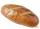# Equation 29

Solve next equation:

2 ( 2x + 3 ) = 8 ( 1 - x) -5 ( x -2 )

Result

x =  0.706

#### Solution:

2 ( 2x + 3 ) = 8 ( 1 - x) -5 ( x -2 )

17x = 12

x = 1217 ≈ 0.705882

Calculated by our simple equation calculator.

Leave us a comment of example and its solution (i.e. if it is still somewhat unclear...):

Showing 0 comments:Be the first to comment!#### To solve this verbal math problem are needed these knowledge from mathematics:

Do you have a linear equation or system of equations and looking for its solution? Or do you have quadratic equation?

## Next similar examples:

1. Find xSolve: if 2(x-1)=14, then x= (solve an equation with one unknown)
2. Simple equationSolve for x: 3(x + 2) = x - 18
3. Simple equationSolve the following simple equation: 2. (4x + 3) = 2-5. (1-x)
4. NormThree workers planted 3555 seedlings of tomatoes in one dey. First worked at the standard norm, the second planted 120 seedlings more and the third 135 seedlings more than the first worker. How many seedlings were standard norm?
5. Dropped sheetsThree consecutive sheets dropped from the book. The sum of the numbers on the pages of the dropped sheets is 273. What number has the last page of the dropped sheets?
6. Unknown number 11That number increased by three equals three times itself?
7. Forest nurseryIn the forest nursery after winter, they found that 1/10 stems died out of them. For them, they land 193 new spruces. How many spruces are in the forest nursery?
8. Negative in equation2x + 3 + 7x = – 24, what is the value of x?
9. TreesFrom the total number of trees in the orchard, there are two-fifths pearls and apples are three eighty. The rest of the trees are 9 ceremonial. How many trees are in the set?
10. Unknown numberI think the number. I'll reduce it to its one-third. The result is then increased by one-third, and I get the number 12.
11. Grandmother and grandfatherGrandmother baked cakes. Grandfather ate half, then quarter of the rest ate Peter and Paul ate half of rest. For parents left 6 cakes. How many cakes maked the grandmother?
12. NumberI think the number. If I add to its third seven I get same as when to its quarter add 8. Which is the number?
13. Number with onesThe first digit of the number is 1, if we move this digit to the end we get a 3 times higher number, which is the number?
14. Equation?
15. How oldThe student who asked how many years he answered: "After 10 years I will be twice as old than as I was four years ago. How old is student?
16. Bread5 of the same bread has the same weight as three bread and 4 kg of fruit. What weight has one bread?
17. Tickets 3A total of 645 tickets were sold for the school play. They were either adult tickets or student tickets. There were 55 fewer student tickets sold than adult tickets. How many adult tickets were sold?